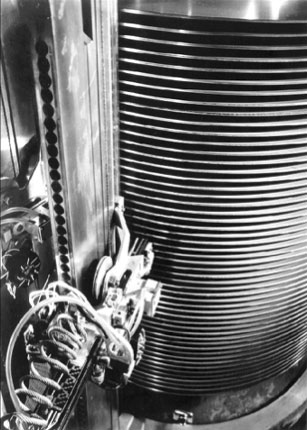Search IntMath
Close

# The hard disk drive is 50 years old

By Murray Bourne, 10 Oct 2006

This monster is the first HDD, released in 1956 and known as the RAMAC.

[It] stored only 5 megabytes of data on 50 disks, 24 inches in diameter. It weighed more than 250 kilograms.Be the first to comment below.

### Comment Preview

HTML: You can use simple tags like <b>, <a href="...">, etc.

To enter math, you can can either:

1. Use simple calculator-like input in the following format (surround your math in backticks, or qq on tablet or phone):
a^2 = sqrt(b^2 + c^2)
(See more on ASCIIMath syntax); or
2. Use simple LaTeX in the following format. Surround your math with $$ and $$.
$$\int g dx = \sqrt{\frac{a}{b}}$$
(This is standard simple LaTeX.)

NOTE: You can mix both types of math entry in your comment.